islocalmax

Find local maxima

Description

example

TF = islocalmax(A) returns a logical array whose elements are 1 (true) when a local maximum is detected in the corresponding element of an array, table, or timetable.

TF = islocalmax(A,dim) specifies the dimension of A to operate along. For example, islocalmax(A,2) finds local maxima of each row of a matrix A.

example

TF = islocalmax(___,Name,Value) specifies additional parameters for finding local maxima using one or more name-value pair arguments. For example, islocalmax(A,'SamplePoints',t) finds local maxima of A with respect to the time stamps contained in the time vector t.

example

[TF,P] = islocalmax(___) also returns the prominence corresponding to each element of A for any of the previous syntaxes.

Examples

collapse all

Compute and plot the local maxima of a vector of data.

x = 1:100;
A = (1-cos(2*pi*0.01*x)).*sin(2*pi*0.15*x);
TF = islocalmax(A);
plot(x,A,x(TF),A(TF),'r*')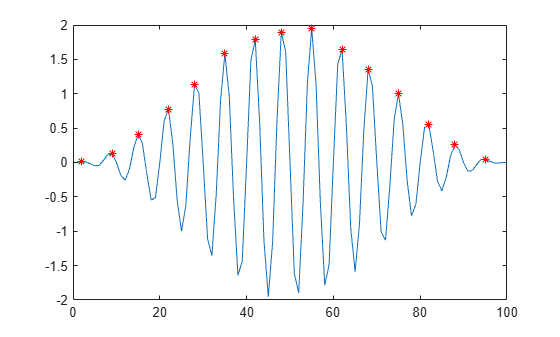Create a matrix of data, and compute the local maxima for each row.

A = 25*diag(ones(5,1)) + rand(5,5);
TF = islocalmax(A,2)
TF = 5x5 logical array

0   0   1   0   0
0   1   0   0   0
0   0   1   0   0
0   1   0   1   0
0   1   0   0   0

Compute the local maxima of a vector of data relative to the time stamps in the vector t. Use the 'MinSeparation' parameter to compute maxima that are at least 45 minutes apart.

t = hours(linspace(0,3,15));
A = [2 4 6 4 3 7 5 6 5 10 4 -1 -3 -2 0];
TF = islocalmax(A,'MinSeparation',minutes(45),'SamplePoints',t);
plot(t,A,t(TF),A(TF),'r*')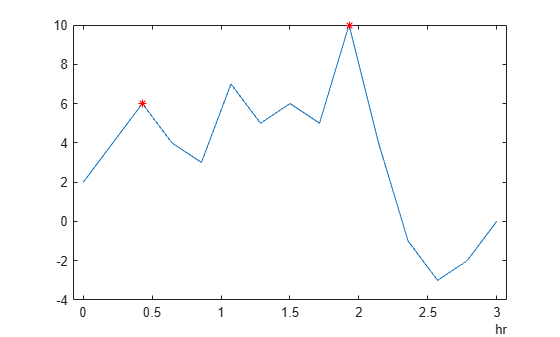Specify a method for indicating consecutive maxima values.

Compute the local maxima of data that contains consecutive maxima values. Indicate the maximum of each flat region based on the first occurrence of that value.

x = 0:0.1:5;
A = min(0.75, sin(pi*x));
TF1 = islocalmax(A, 'FlatSelection', 'first');
plot(x,A,x(TF1),A(TF1),'r*')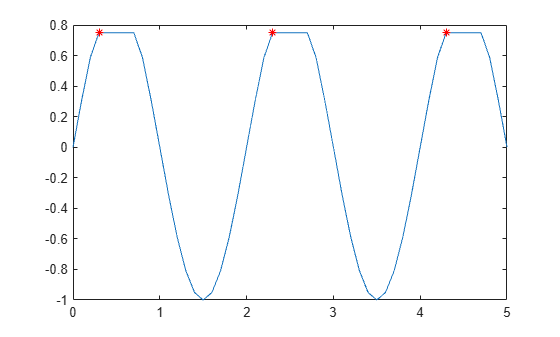Indicate the maximum of each flat region with all occurrences of that value.

TF2 = islocalmax(A, 'FlatSelection', 'all');
plot(x,A,x(TF2),A(TF2),'r*')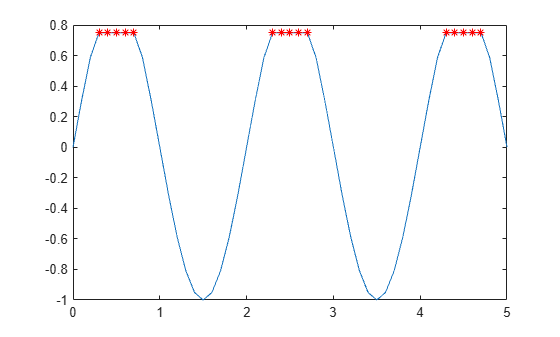Select maxima based on their prominence.

Compute the local maxima of a vector of data and their prominence, and then plot them with the data.

x = 1:100;
A = peaks(100);
A = A(50,:);
[TF1,P] = islocalmax(A);
P(TF1)
ans = 1×2

1.7703    3.5548

plot(x,A,x(TF1),A(TF1),'r*')
axis tight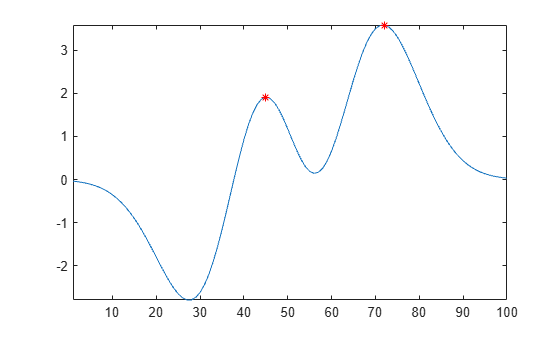Compute only the most prominent maximum in the data by specifying a minimum prominence requirement.

TF2 = islocalmax(A,'MinProminence',2);
plot(x,A,x(TF2),A(TF2),'r*')
axis tight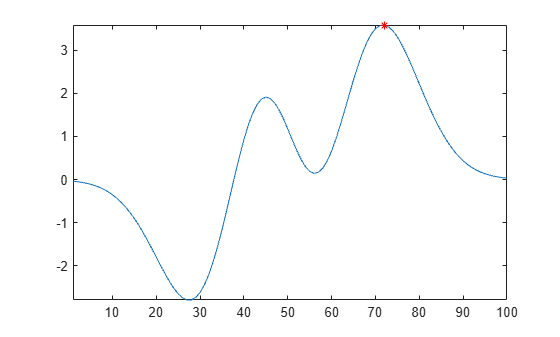Input Arguments

collapse all

Input data, specified as a vector, matrix, multidimensional array, table, or timetable.

Data Types: single | double | int8 | int16 | int32 | int64 | uint8 | uint16 | uint32 | uint64 | logical | table | timetable

Operating dimension, specified as a positive integer scalar. By default, islocalmax operates along the first dimension whose size does not equal 1.

For example, if A is a matrix, then islocalmax(A,1) operates along the rows of A, computing local maxima for each column.islocalmax(A,2) operates along the columns of A, computing local maxima for each row.Data Types: single | double | int8 | int16 | int32 | int64 | uint8 | uint16 | uint32 | uint64

Name-Value Arguments

Specify optional comma-separated pairs of Name,Value arguments. Name is the argument name and Value is the corresponding value. Name must appear inside quotes. You can specify several name and value pair arguments in any order as Name1,Value1,...,NameN,ValueN.

Example: TF = islocalmax(A,'MinProminence',2)
Data Options

collapse all

Sample points, specified as the comma-separated pair consisting of 'SamplePoints' and either a vector of sample point values or one of the options in the following table when the input data is a table. The sample points represent the x-axis locations of the data, and must be sorted and contain unique elements. Sample points do not need to be uniformly sampled. The vector [1 2 3 ...] is the default.

When the input data is a table, you can specify the sample points as a table variable using one of the following options.

Option for Table InputDescriptionExamples
Variable name

A character vector or scalar string specifying a single table variable name

'Var1'

"Var1"

Scalar variable index

A scalar table variable index

3

Logical vector

A logical vector whose elements each correspond to a table variable, where true specifies the corresponding variable as the sample points, and all other elements are false

[true false false]

Function handle

A function handle that takes a table variable as input and returns a logical scalar, which must be true for only one table variable

@isnumeric

vartype subscript

A table subscript generated by the vartype function that returns a subscript for only one variable

vartype('numeric')

Note

This name-value pair is not supported when the input data is a timetable. Timetables always use the vector of row times as the sample points. To use different sample points, you must edit the timetable so that the row times contain the desired sample points.

Example: islocalmax(A,'SamplePoints',0:0.1:10)

Example: islocalmax(T,'SamplePoints',"Var1")

Data Types: double | single | int8 | int16 | int32 | int64 | uint8 | uint16 | uint32 | uint64 | datetime | duration

Table variables to operate on, specified as the comma-separated pair consisting of 'DataVariables' and one of the options in this table. The 'DataVariables' value indicates which variables of the input table to examine for local maxima. The data type associated with the indicated variables must be numeric or logical. Other variables in the table not specified by 'DataVariables' are not operated on, so the output contains false values for those variables.

OptionDescriptionExamples
Variable name

A character vector or scalar string specifying a single table variable name

'Var1'

"Var1"

Vector of variable names

A cell array of character vectors or string array where each element is a table variable name

{'Var1' 'Var2'}

["Var1" "Var2"]

Scalar or vector of variable indices

A scalar or vector of table variable indices

1

[1 3 5]

Logical vector

A logical vector whose elements each correspond to a table variable, where true includes the corresponding variable and false excludes it

[true false true]

Function handle

A function handle that takes a table variable as input and returns a logical scalar

@isnumeric

vartype subscript

A table subscript generated by the vartype function

vartype('numeric')

Example: islocalmax(T,'DataVariables',["Var1" "Var2" "Var4"])

Extrema Detection Options

collapse all

Minimum prominence, specified as the comma-separated pair consisting of 'MinProminence' and a nonnegative scalar. islocalmax returns only local maxima whose prominence is at least the value specified.

Data Types: single | double | int8 | int16 | int32 | int64 | uint8 | uint16 | uint32 | uint64

Prominence window, specified as the comma-separated pair consisting of 'ProminenceWindow' and a positive integer scalar, a two-element vector of positive integers, a positive duration scalar, or a two-element vector of positive durations. The value defines a window of neighboring points for which to compute the prominence for each local maximum.

When the window value is a positive integer scalar k, then the window is centered about each local maximum and contains k-1 neighboring elements. If k is even, then the window is centered about the current and previous elements. If a local maximum is within a flat region, then islocalmax treats the entire flat region as the center point of the window.

When the value is a two-element vector [b f] of positive integers, then the window contains the local maximum, b elements backward, and f elements forward. If a local maximum is within a flat region, then the window starts b elements before the first point of the region and ends f elements after the last point of the region.

When the input data is a timetable or when 'SamplePoints' is specified as a datetime or duration vector, the window value must be of type duration, and the window is computed relative to the sample points.

Data Types: single | double | int8 | int16 | int32 | int64 | uint8 | uint16 | uint32 | uint64 | duration

Flat region indicator for when a local maximum value is repeated consecutively, specified as the comma-separated pair consisting of 'FlatSelection' and one of the following:

• 'center' — Indicate only the center element of a flat region as the local maximum. The element of TF corresponding to the center of the flat is 1, and is 0 for the remaining flat elements.

• 'first' — Indicate only the first element of a flat region as the local maximum. The element of TF corresponding to the start of the flat is 1, and is 0 for the remaining flat elements.

• 'last' — Indicate only the last element of a flat region as the local maximum. The element of TF corresponding to the end of the flat is 1, and is 0 for the remaining flat elements.

• 'all' — Indicate all the elements of a flat region as the local maxima. The elements of TF corresponding to all parts of the flat are 1.

When using the 'MinSeparation' or 'MaxNumExtrema' name-value pairs, flat region points are jointly considered a single maximum point.

Minimum separation between local maxima, specified as the comma-separated pair consisting of 'MinSeparation' and a nonnegative scalar. The separation value is defined in the same units as the sample points vector, which is [1 2 3 ...] by default. When the separation value is greater than 0, islocalmax selects the largest local maximum and ignores all other local maxima within the specified separation. This process is repeated until there are no more local maxima detected.

When the sample points vector has type datetime, the separation value must have type duration.

Data Types: single | double | int8 | int16 | int32 | int64 | uint8 | uint16 | uint32 | uint64 | duration

Maximum number of maxima detected, specified as the comma-separated pair consisting of 'MaxNumExtrema' and a positive integer scalar. islocalmax finds no more than the specified number of most prominent maxima, which is the length of the operating dimension by default.

Data Types: single | double | int8 | int16 | int32 | int64 | uint8 | uint16 | uint32 | uint64

Output Arguments

collapse all

Local maxima indicator, returned as a vector, matrix, or multidimensional array. TF is the same size as A.

Data Types: logical

Prominence, returned as a vector, matrix, or multidimensional array. P is the same size as A.

If the input data has a signed or unsigned integer type, then P is an unsigned integer.

collapse all

Prominence of Local Maximum

The prominence of a local maximum (or peak) measures how the peak stands out with respect to its height and location relative to other peaks.

To measure the prominence of a peak, first extend a horizontal line from the peak to the left and to the right of the peak. Find where the line intersects the data on the left and on the right, which will either be another peak or the end of the data. Mark these locations as the outer endpoints of the left and right intervals. Next, find the lowest valley in both intervals. Take the larger of these two valleys, and measure the vertical distance from that valley to the peak. This distance is the prominence.

For a vector x, the largest prominence is at most max(x)-min(x).